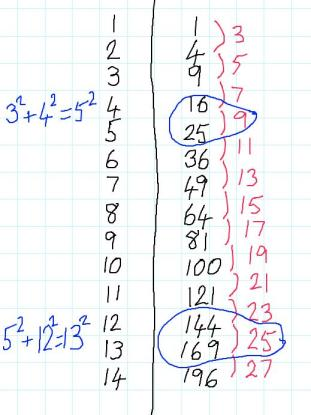#### You may also like### Odd Differences

The diagram illustrates the formula: 1 + 3 + 5 + ... + (2n - 1) = nÂ² Use the diagram to show that any odd number is the difference of two squares.### Triangular Triples

Show that 8778, 10296 and 13530 are three triangular numbers and that they form a Pythagorean triple.# Generating Triples

##### Age 14 to 16Challenge Level

Charlie has been investigating square numbers. He decided to organise his work in a table:Charlie noticed some special relationships between certain square numbers:

$$3^2+4^2=5^2$$ $$5^2+12^2=13^2$$

Sets of integers like ${3,4,5}$ and ${5,12,13}$ are called Pythagorean Triples, because they could be the lengths of the sides of a right-angled triangle.

He wondered whether he could find any more...

Can you extend Charlie's table to find any more sets of Pythagorean Triples where the hypotenuse is 1 unit longer than one of the other sides?
Do you notice any patterns?
Can you make any predictions?

Can you find a formula that generates Pythagorean Triples like Charlie's?
Can you prove that your formula works?

Alison has been working on Pythagorean Triples where the hypotenuse is 2 units longer than one of the other sides.
So far, she has found these:
$$4^2 + 3^2 = 5^2$$ $$6^2+8^2=10^2$$ $$8^2+15^2=17^2$$

Some of these are just scaled-up versions of Charlie's triples, but some of them are new and can't be divided by a common factor (these are called primitive triples).

Can you find more Pythagorean Triples like Alison's?

Can you find a formula for generating Pythagorean Triples like Alison's?
Can you prove that your formula works?

Here are some follow-up questions you might like to consider:

• Can you find Triples where the hypotenuse is 3 units longer than one of the other sides? Or 4 units longer? Or...?
• Can you say anything about when such triples will be primitive triples?

For a challenging extension investigation, why not take a look at Few and Far Between?